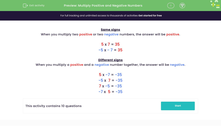Multiply Positive and Negative Numbers

In this worksheet, students practise multiplying together positive and negative numbers.Key stage:  KS 3

Curriculum topic:   Number

Curriculum subtopic:   Use Four Operations for All Numbers

Difficulty level:Worksheet Overview

Same signs

When you multiply two positive or two negative numbers, the answer will be positive.

5 x 7 = 35

-5 x - 7 = 35

Different signs

When you multiply a positive and a negative number together, the answer will be negative.

5 x -7 = -35

-5 7 = -35

7 x -5 = -35

-7 5 = -35

What is EdPlace?

We're your National Curriculum aligned online education content provider helping each child succeed in English, maths and science from year 1 to GCSE. With an EdPlace account you’ll be able to track and measure progress, helping each child achieve their best. We build confidence and attainment by personalising each child’s learning at a level that suits them.

Get started